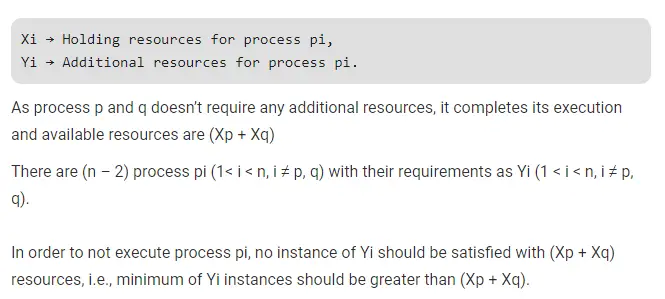# Gate CS-2019 Question Paper With Solutions

Q. 48 Consider the following snapshot of a system running n concurrent processes. Process i is holding Xi instances of a resource R, 1 ≤ i ≤ n. Assume that all instances of R arecurrently in use. Further, for all i, process i can place a request for at most Yi additional instances of R while holding the Xt instances it already has. Of the n processes, there are exactly two processes p and q such that Yp = Yq = 0.

Which one of the following conditions guarantees that no other process apart from p and q can complete execution?

(A) Xp + Xq < Min {Yk ⏐ 1 ≤ k ≤ n, k ≠ p, k ≠ q}

(B) Min (Xp, Xq) ≥ Min {Yk ⏐ 1 ≤ k≤ n, k ≠ p, k ≠ q}

(C) Min (Xp, Xq) ≤ Max {Yk ⏐ 1 ≤ k ≤ n, k ≠ p, k ≠ q}

(D) Xp + Xq < Max {Yk ⏐ 1 ≤ k ≤ n, k ≠ p, k ≠ q}